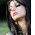Welcome! Dear Traders,you are reading my forex trading experiences. Forex trading is a very profitable and very risky business opportunity. If you are a beginner, calm down,have a cup of coffee, and convince yourself that you need to study hard to win in forex trading. Obviously, the task is not easy as the statistics claim that only 5% traders win in forex trading. If you are determined, serious,and hard working, you can surely be included in the group of winners.

## Sunday, January 11, 2015

### WHAT ARE PIP AND PIPETTE

A pip is a unit of measurement that expresses the changes in the value of a currency. Pipette is smaller unit of pip, 1 pip = 10 pipette. Most of the traders calculate their gain and loss with pipette, but pip is the widely accepted unite to express the changes in the value of a currency. This post explains "What is a pip?", "What is a pipette?", and "How to calculate the value of a pip and a pipette?"

Let's calculate the value of a pip and a pipette of EUR/USD. Let the price of EUR/USD at certain time is 1.2548, it means 1EUR=1.2548USD. We know in the EUR/USD currency pair, EUR is the base currency and USD is the counter currency, and the quotes of a currency pair express the value of base currency in terms of counter currency.

The formula to calculate pip value is - Pip value = the value change in counter currency times the exchange rate ratio, and the formula to calculate pipette value is Pipette value = pip value per unite/10 Let the price of EUR/USD moved to 1.2549 from 1.2548, it means the price of EUR/USD moved 1pip higher.

So here, the value change in counter currency= 0.0001, and exchange rate ratio=1/1.2549. So, pip value per unite = 0.0001X1/1.2549 = 0.0001/1.2549 = 0.000080USD, and Pipette value per unite = pip value per unite/10 = 0.000080/10 = 0.0000080USD.

In the example above pip value per unit and pipette value per unit are calculated. But how the pip value and pipette value will be calculated for a trade of 10,000 unit of a currency. The technique is simple just multiply the per unit pip value and per unit pipette value by 10,000.

So the final formula to calculate the pip value is Pip value = the value change in counter currency times the exchange rate ratio times the unit of currency traded. And the final formula to calculate the pipette value is Pipette value = the value change in counter currency times the exchange rate ratio times the unit of currency traded/10

Generally, the standard form of currency quotes have 4 or 2 digits after the decimals, but some brokers offer currency quotes that have 5 digits or 3 digits after the decimal. In case of standard form of currency quotes, the last digit after the decimal represents pips, and here pipette is not in use. In the modern form of currency quotes the second digit from the right hand side represents the pips and the first digit from the right hand side represents the pipettes.

To calculate the pips value of a currency pair quoted in 5 or 3 digits form, you can leave the last digit after the decimal.In another way, the pipette value can be directly calculated from the quote with 5 digits after the decimal, and then it can be multiplied by 10 to calculate the pip value. The pip and pipette value of the currency quoted in 5 or 3 digits form can be calculated with following formulas.

Pipette value = the value change in counter currency times the exchange rate ratio times the unit of currency traded
Pip value = the value change in counter currency times the exchange rate ratio times the unit of currency traded X 10

Dear Traders, If you are still struggling to calculate the pip and pipette value, just drop a comment below. I will response you as early as possible. Two remarkable applications of pip and pipette value are to calculate the cost of an order and to calculate the total profit and loss.

1.Good day, i am trying to understand the calculations of pip and pipettes,but i am still struggling. from: Shirley Botha

1.Apply the formulas and calculate the pipette value of certain currency pair . It would be better for me to answer, if you ask me specifically which point you did not understand.

2.I like their forex-copy system. You can copy the trades of successful traders and earn money even if you're newbie.

3.